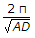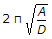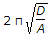# Civil Engineering - UPSC Civil Service Exam Questions

1.

The moment of inertia of a given rectangular area is minimum about

 A. its longer centroidal axis B. its polar axis C. its axis along the diagonal D. its shorter centroidal axis

Explanation:

No answer description available for this question. Let us discuss.

2.

If the efficiencies of BOD removal of first-stage and second-stage trickling filters are each 65.0%, then what is the overall BOD removal efficiency of these filters ?

 A. 65% B. 77.25% C. 87.75% D. 92.6%

Explanation:

No answer description available for this question. Let us discuss.

3.

Which one of the following tests can be carried out at the construction site to determine the dynamic elastic properties of soil ?

 A. Block vibration test B. Dutch cone penetration test C. Standard penetration test D. Vane shear test

Explanation:

No answer description available for this question. Let us discuss.

4.

A particle moves with simple harmonic motion. If its acceleration at distance 'D' from the equilibrium position is 'A' then the period of the motion is given by

 A. 2πAD B.C.D.Explanation:

No answer description available for this question. Let us discuss.

5.

Consider the following statements :
A high lime content in a composite cement-lime mortor results in
1. slow hardening.
2. quick setting.
3. weaker mortar.
Of these statements :

 A. 2 and 3 are correct B. 1 and 2 are correct C. 1 and 3 are correct D. 1, 2 and 3 are correct